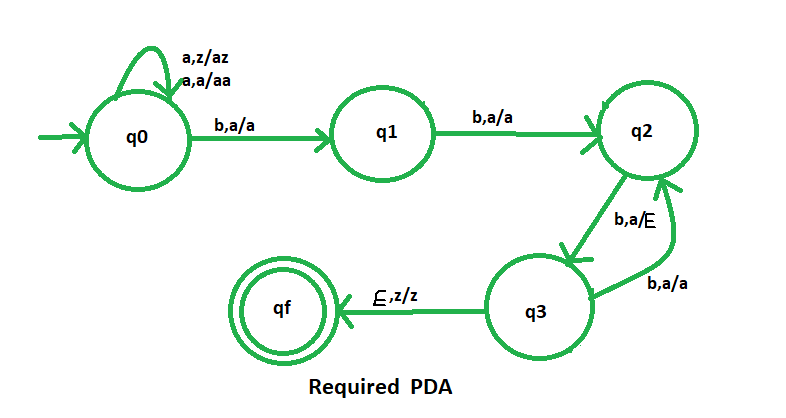# NPDA for accepting the language L = {amb(2m+1) | m ≥ 1}

• Difficulty Level : Hard
• Last Updated : 15 Jun, 2022

Prerequisite – Pushdown automata, Pushdown automata acceptance by final state

Problem – Design a non deterministic PDA for accepting the language L = {| m ≥ 1}, or,L = {| m ≥ 1}, i.e.,

L = {abbb, aabbbbb, aaabbbbbbb, aaaabbbbbbbbb, ......}

In each of the string, the number of ‘b’ is one more than the twice of the number of ‘a’.

Explanation – Here, we need to maintain the order of a’s and b’s.That is, all the a’s  are coming first and then all the b’s are coming. Thus, we need a stack along with the state diagram. The count of a’s and b’s is maintained by the stack. We will take 2 stack alphabets:= { a, z }Where,= set of all the stack alphabetz = stack start symbol

Approach used in the construction of PDA – As we want to design a NPDA, thus every time ‘a’ comes before ‘b’. We will push one ‘a’ into the stack for every ‘a’s and again for the next ‘a’, we will push one ‘a’ into the stack. And then when ‘b’ comes, for the first ‘b’ we will do nothing only state will change. For the next two ‘b’ we will pop one ‘a’ and again for next two ‘b’, we pop one ‘a’. And similarly we perform this alternatively. i.e., For third ‘b’ we pop first ‘a’ For fifth ‘b’ we pop second ‘a’ For seventh ‘b’ we pop third ‘a’ So, at the end if the stack becomes empty then we can say that the string is accepted by the PDA.

Stack transition functions –(q0, a, z)(q0, az)(q0, a, a)(q0, aa)(q0, b, a)(q1, a)  [ Indicates no operation only state change ](q1, b, a)(q2, a)  [ Indicates no operation only state change ](q2, b, a)(q3,)  [Indicates pop operation ](q3, b, a)(q2, a )  [ Indicates no operation only state change ](q3,, z)(qf, z )

Where, q0 = Initial state qf = Final state= indicates pop operationMy Personal Notes arrow_drop_up